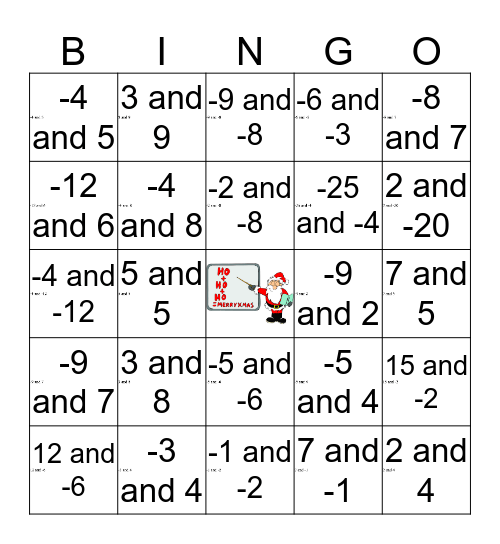# That's Sum ProductThis bingo card has 24 images, a free space and 24 words: 3 and 8, -3 and 4, -2 and -8, 12 and -6, -8 and 7, -4 and 5, -12 and 6, 3 and 9, -9 and 2, -5 and -6, -25 and -4, -4 and -12, -5 and 4, 15 and -2, -4 and 8, 7 and -1, 7 and 5, -9 and -8, 2 and -20, 5 and 5, 2 and 4, -9 and 7, -6 and -3 and -1 and -2.

## Play Online